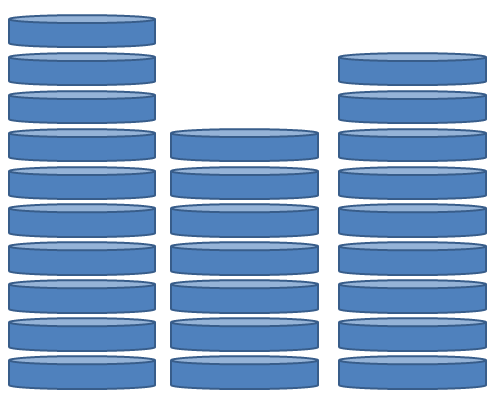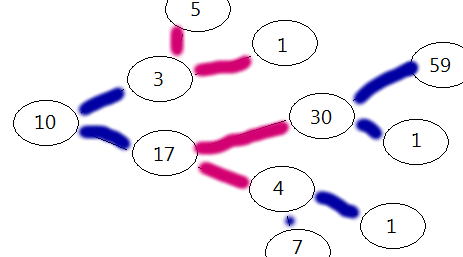Probability

# Combinatorial Games: Level 4 ChallengesTwo players play a game according to the following rules:

• They place three heaps of coins on a table. The first heap has $10$ coins, the second heap has $7$ coins, and the third heap has $9$ coins.

• The second player adds another pile of coins on the table, having at most $10$ coins.

• The players take turns alternately, starting with the first player. At each move, the player has to remove a positive number of coins from one heap. The player who removes the last coin wins.

It turns out that regardless of the strategy of the first player, the second player always wins with optimal play. How many coins should the second player add in the fourth pile?There are $n$ pirates $\left(P_{n}, P_{n-1}, \cdots P_1 \right)$ with the strict order of superiority $\left(P_{n} > P_{n-1} > \cdots > P_1 \right)$.

They find 100 gold coins. They must distribute the coins among themselves according to the following rules:

1. The superior-most surviving pirate proposes a distribution.
2. The pirates, including the proposer, then vote on whether to accept this distribution.
3. In case of a tie vote the proposer has the casting vote.
4. If the distribution is accepted, the coins are disbursed and the game ends. If not, the proposer is thrown overboard from the pirate ship and dies (leaving behind $(n-1)$ pirates and their original ordering), and the next most superior pirate makes a new proposal to begin the game again.

Every pirate knows that every other pirates is perfectly rational, which means:

1. They will always be able to deduce something logically deducible.
2. Their first preference is that they want to survive.
3. Their second preference is that they want to maximize the number of coins they receive.
4. Other things remaining same, they prefer to kill a pirate rather than having him alive.

What is the minimum value of $n$ such that the superior-most pirate can propose no distribution of coins that will let him survive?Ivan and Jake are playing a game with the following rules:

1. The game starts with a parameter N which is a positive integer.
2. Ivan always plays first.
3. In each turn, the player can either choose to add or subtract the largest prime smaller than N, to N.
4. The loser is the person who cannot continue. The other person wins by default.

Example

 1 2 3 4 5 6 N=10 Ivan: N -> N + 7 = 17 Jake: N -> N - 13 = 4 Ivan: N -> 4 - 3 = 1 Ivan wins because Jake cannot continue the game. 

Now, suppose that Ivan and Jake know how to play optimally. For how many starting values of $N < 10^4$ does Jake win?

Mursalin and Trevor decide to play a game where Trevor gets to pick any positive integer $n<1000$.

After that the game begins.

First Mursalin names an integer $x$ from $1$ to $n$ [both inclusive]. Then Trevor has to name an integer from $1$ to $n$ [both inclusive] that does not divide $x$. Then it's Mursalin's turn again and so on. On each turn the player has to select an integer from $1$ through $n$ [both inclusive] that doesn't divide all the numbers selected so far. The first person unable to name an integer under these constraints loses. Assuming that both players play optimally, for how many $n$'s does Mursalin have a winning strategy?

A two player game is played on a $5 \times 5$ grid. A token starts in the bottom left corner of the grid. On each turn, a player can move the token one or two units to the right, or to the leftmost square of the row immediately above it. The last player who is able to move wins.

Lino and Calvin decide that they want to make the game more interesting and instead of playing with a single token, they will play with two tokens, one red, and one blue, and on a turn a player moves either of the tokens. They also decided that the tokens will start in random positions on the board. Of the $25 \times 25 =625$ possible starting positions for the 2 tokens, how many of these are winning positions for the first player if he plays optimally?

Details and assumptions

Clarification: Both tokens are allowed to be on the same square at any point during the game.

For the edge case where both tokens start in the top right corner, we declare that the second player wins.

×

Problem Loading...

Note Loading...

Set Loading...